# Lions Weekly: October 27, 2021# A Note From Mr. Sandefur

Lion Family,

Good afternoon! I am writing to you today from our first annual college road trip for our juniors and seniors. A group of twenty-four students and three faculty members are visiting six colleges and universities. We are visiting Carson Newman University, University of the Cumberlands, Union University, Tennessee Tech, Middle Tennessee State University, and the University of Tennessee Chattanooga, as we seek to expose students to potential opportunities for the next steps in their educational journey. This group has represented TKA exceptionally well and is looking forward to the remainder of the visits on this trip.

I’d ask that you take some time this week to specifically pray for our juniors and seniors as they seek the Lord’s will for the next steps. We are joining their families in prayer for wisdom, provision, and discernment.

In Christ Alone,

Jeremy Sandefur
President

 table div table+table+table+table div table{width:100%;padding:0}table div table+table+table+table div table img{width:96.23%;padding:0;float:none}table div table+table+table+table div table td{width:100%;padding:0 1.88% 18px}/* styles */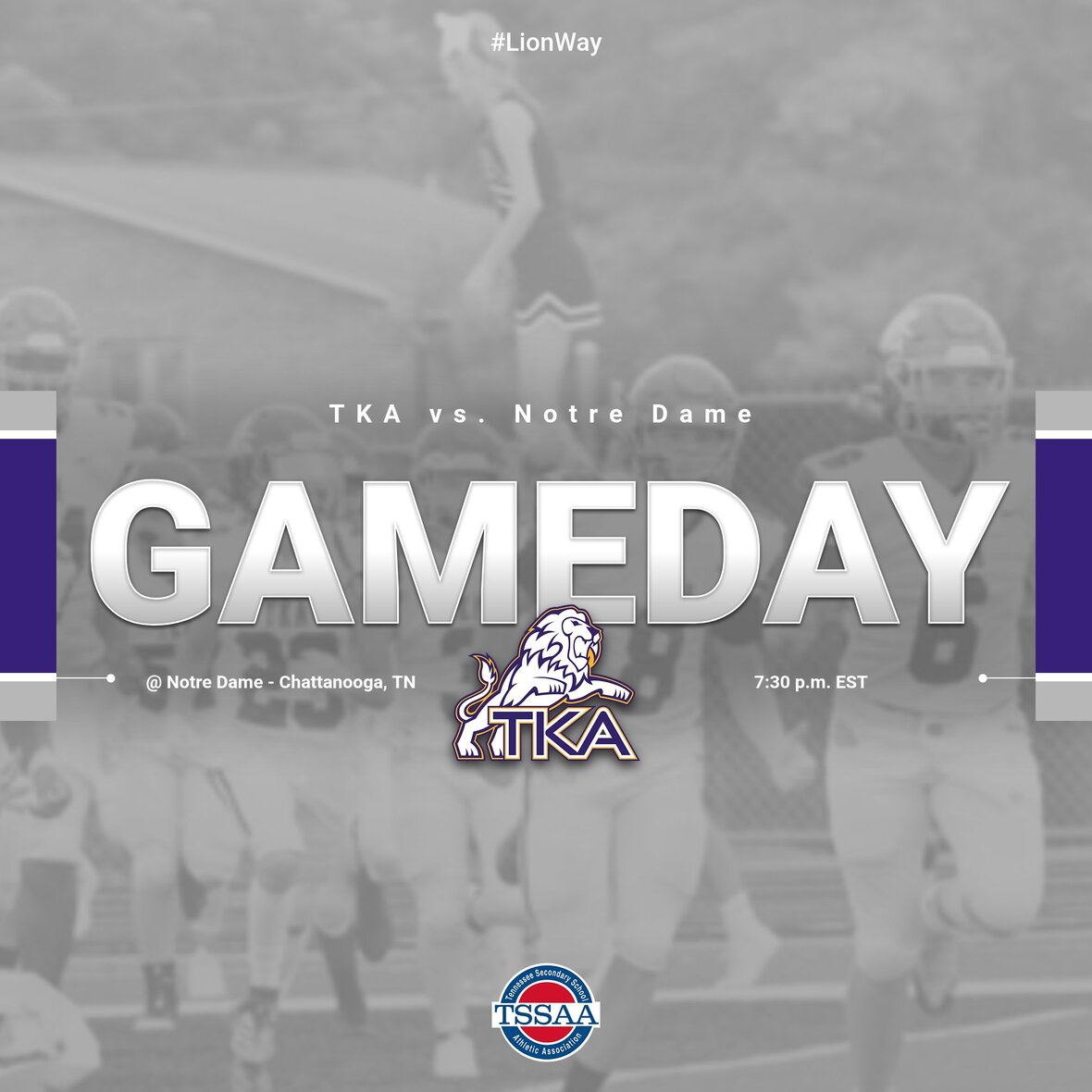# Varsity Football

Congratulations to our Lions on their win over Webb Bell Buckle on Friday, October 22!

TKA will play Notre Dame in Chattanooga, TN, this Friday, October 29. Kickoff is at 7:30 EST. We hope to see you there!

 /* styles */
 table div table+table+table+table+table+table+table div table{width:100%;padding:0}table div table+table+table+table+table+table+table div table img{width:96.23%;padding:0;float:none}table div table+table+table+table+table+table+table div table td{width:100%;padding:0 1.88% 18px}/* styles */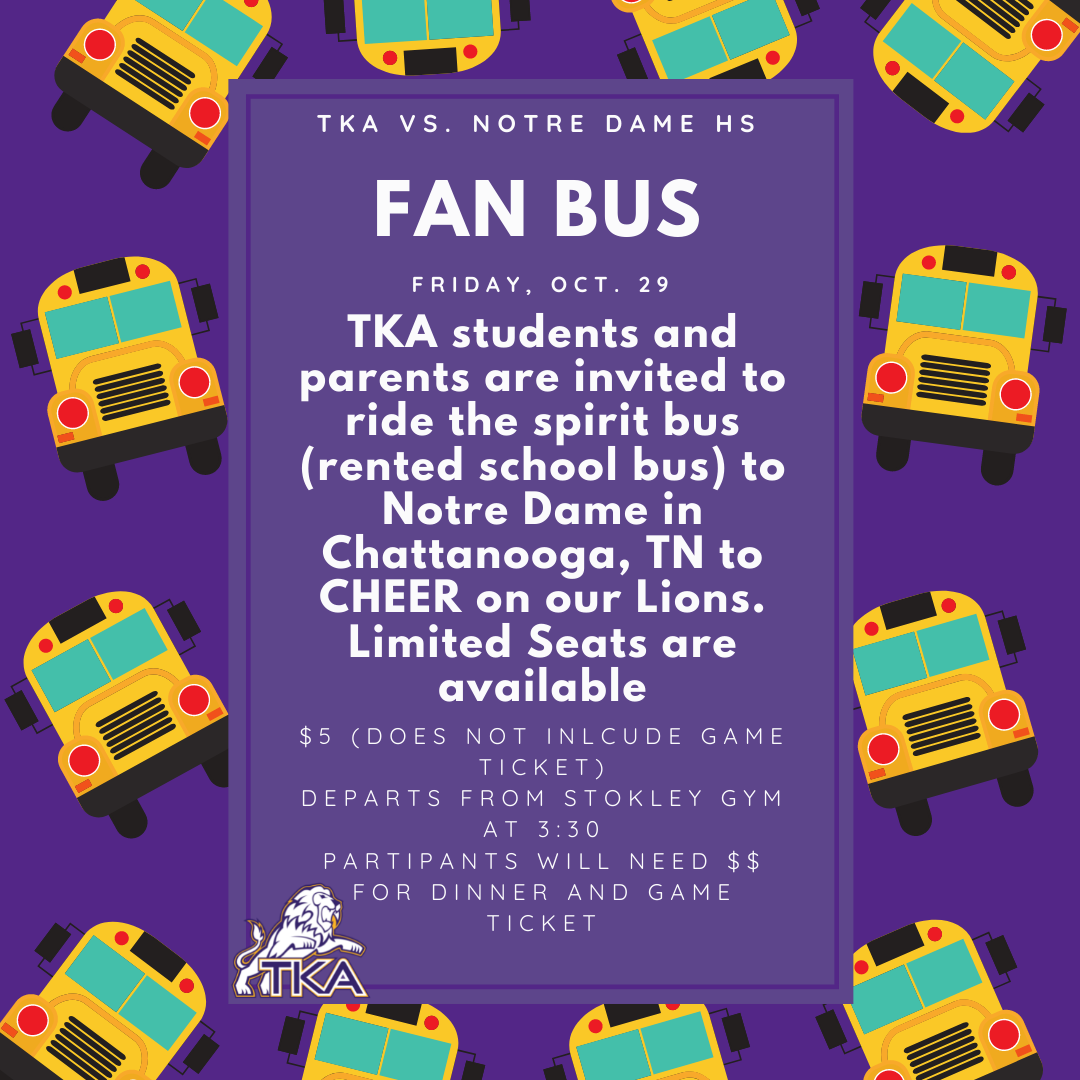# Fan Bus

 table div table+table+table+table+table+table+table+table+table div table{width:100%;padding:0}table div table+table+table+table+table+table+table+table+table div table img{width:96.23%;padding:0;float:none}table div table+table+table+table+table+table+table+table+table div table td{width:100%;padding:0 1.88% 18px}/* styles */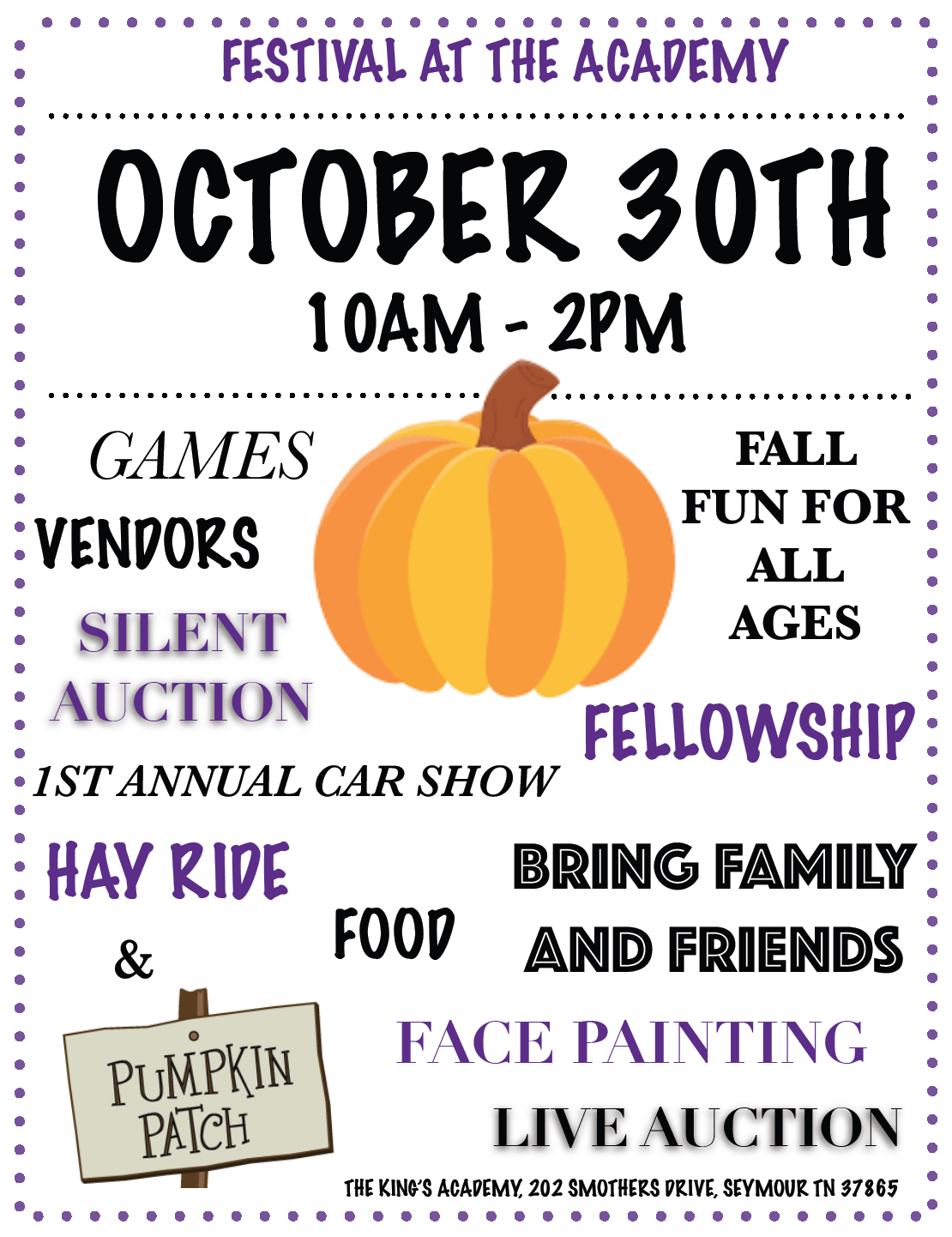We are excited to see everyone at the Festival at The Academy this Saturday, October 30, from 10 a.m. to 2 p.m.

We have 20 vendors, 26 festival games, multiple food vendors, a car show, and so much more!The Academy will also host their first car show on Saturday as well. If you have a car you would like to enter, let us know!

## Contacts for Festival at The Academy:

The King’s Academy (865) 573-8321 ext.5
Kara Pritchard (865) 924-4333
Cherish McLin (865) 604-8303

 table div table+table+table+table+table+table+table+table+table+table+table+table div table{width:100%;padding:0}table div table+table+table+table+table+table+table+table+table+table+table+table div table img{width:96.23%;padding:0;float:none}table div table+table+table+table+table+table+table+table+table+table+table+table div table td{width:100%;padding:0 1.88% 18px}/* styles */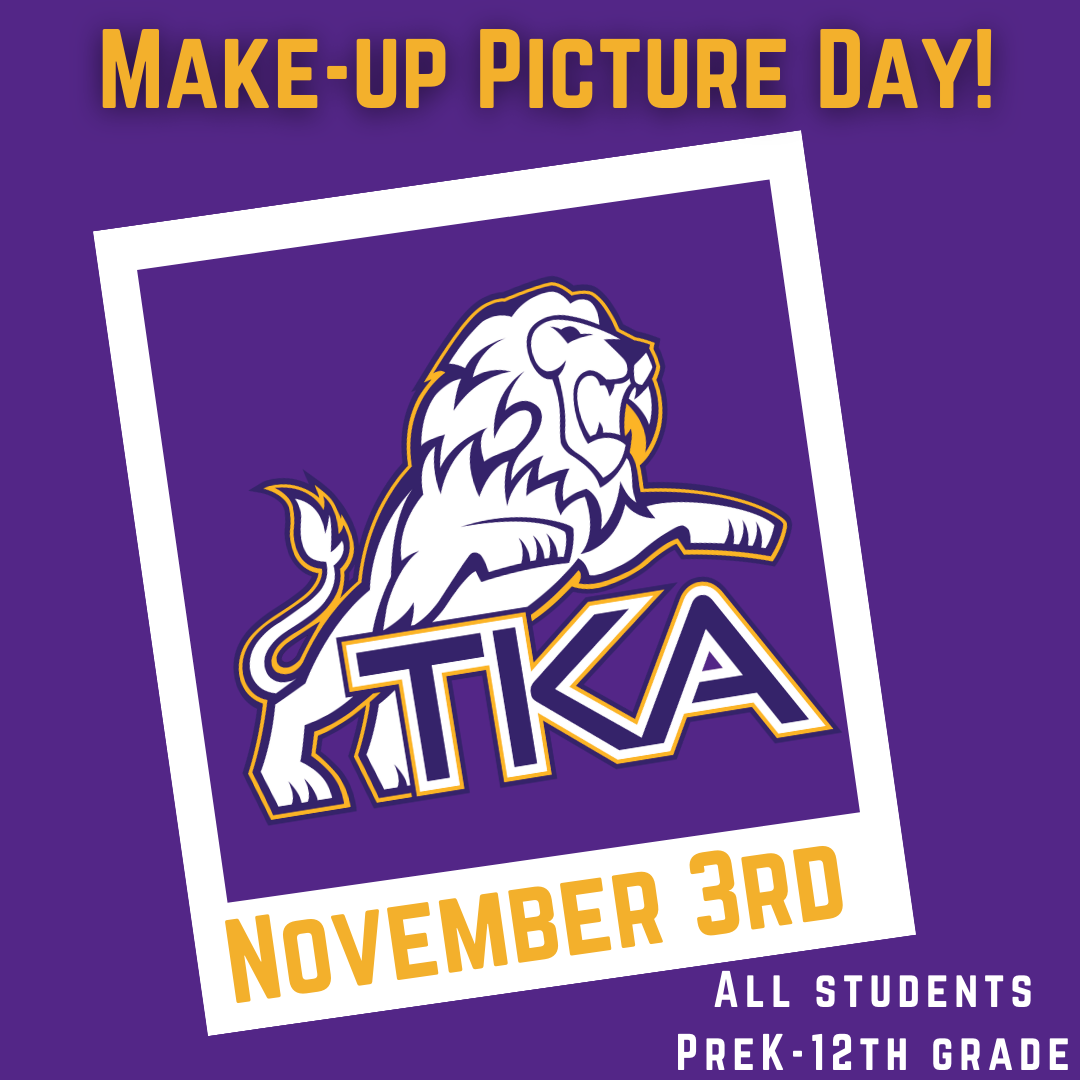# Make-up Picture Day

Anyone who missed taking their yearbook pictures can have their picture made on Wednesday, November 3.

 table div table+table+table+table+table+table+table+table+table+table+table+table+table+table div table{width:100%;padding:0}table div table+table+table+table+table+table+table+table+table+table+table+table+table+table div table img{width:96.23%;padding:0;float:none}table div table+table+table+table+table+table+table+table+table+table+table+table+table+table div table td{width:100%;padding:0 1.88% 18px}/* styles */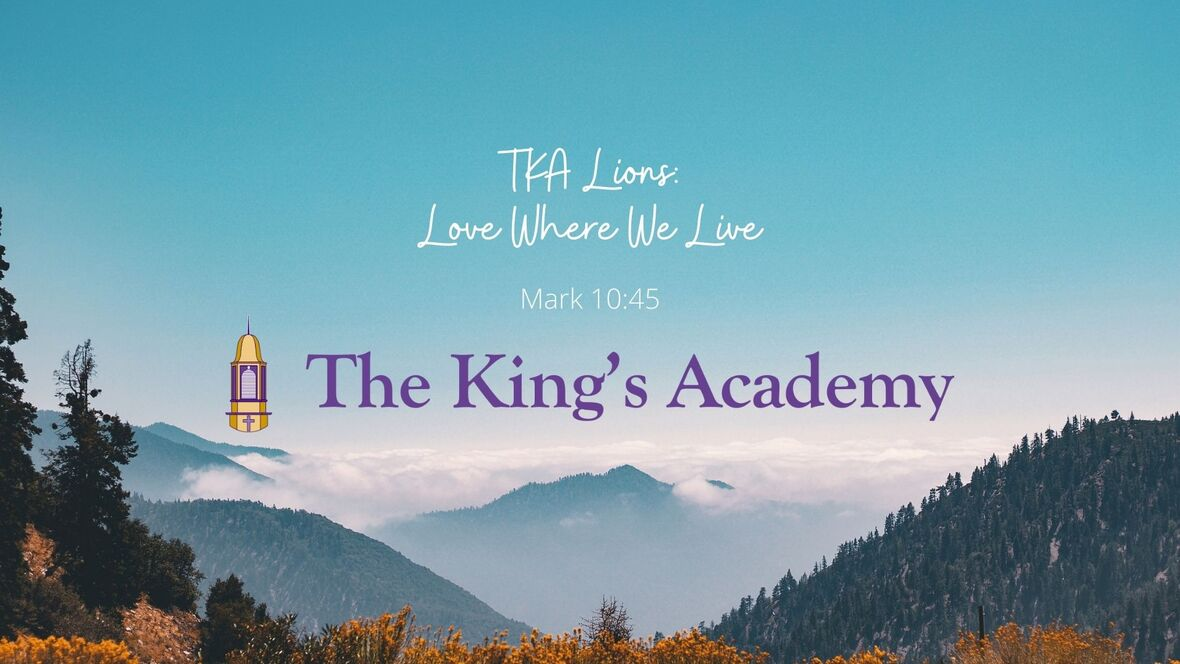# Love Where We Live

TKA Love Where We Live projects are in full swing!

Yesterday, the 9th-grade students participated in their grade level Love Where We Live Project. They washed the ambulances, swept the bays, and cleaned the station for our Seymour EMT station. We are thankful for the opportunity to serve our first responders in the Seymour community!

Our 12th-grade students participated in a Love Where We Love activity by creating encouragement cards for healthcare workers at UT Medical Center. Thank you to all of our health care workers who continue to take care of those in need!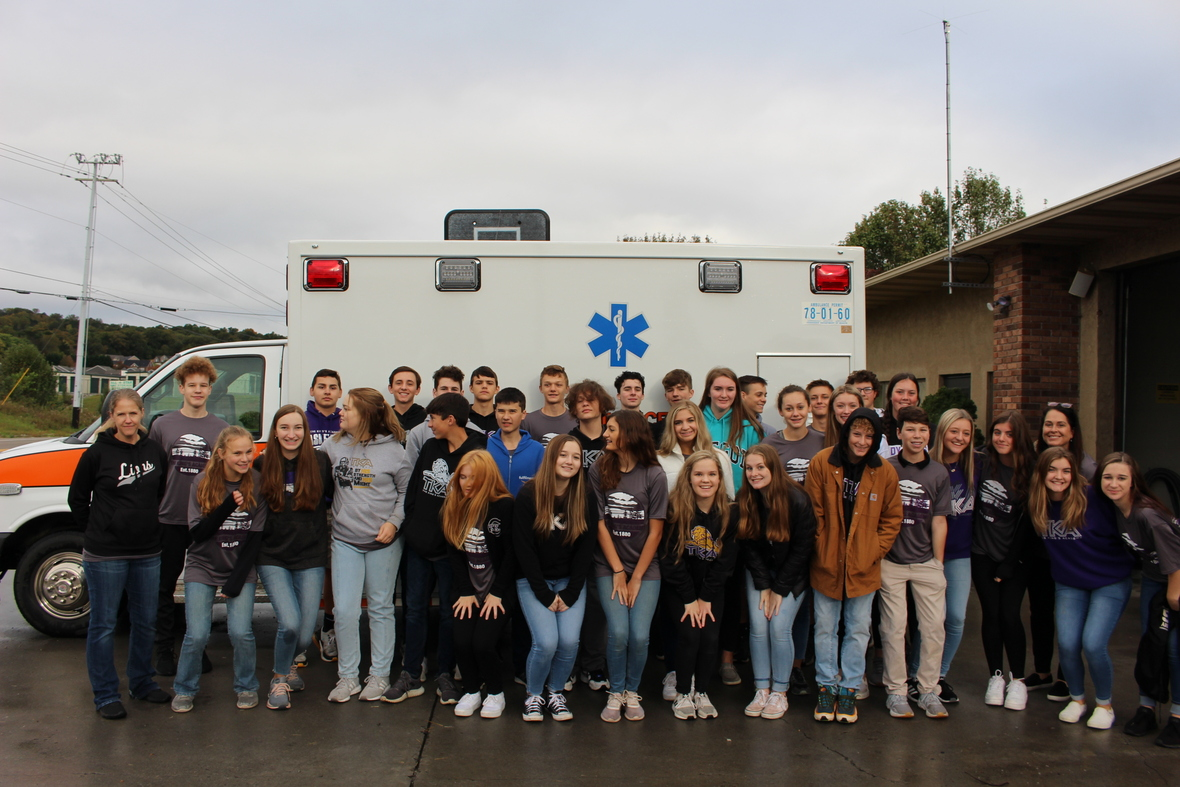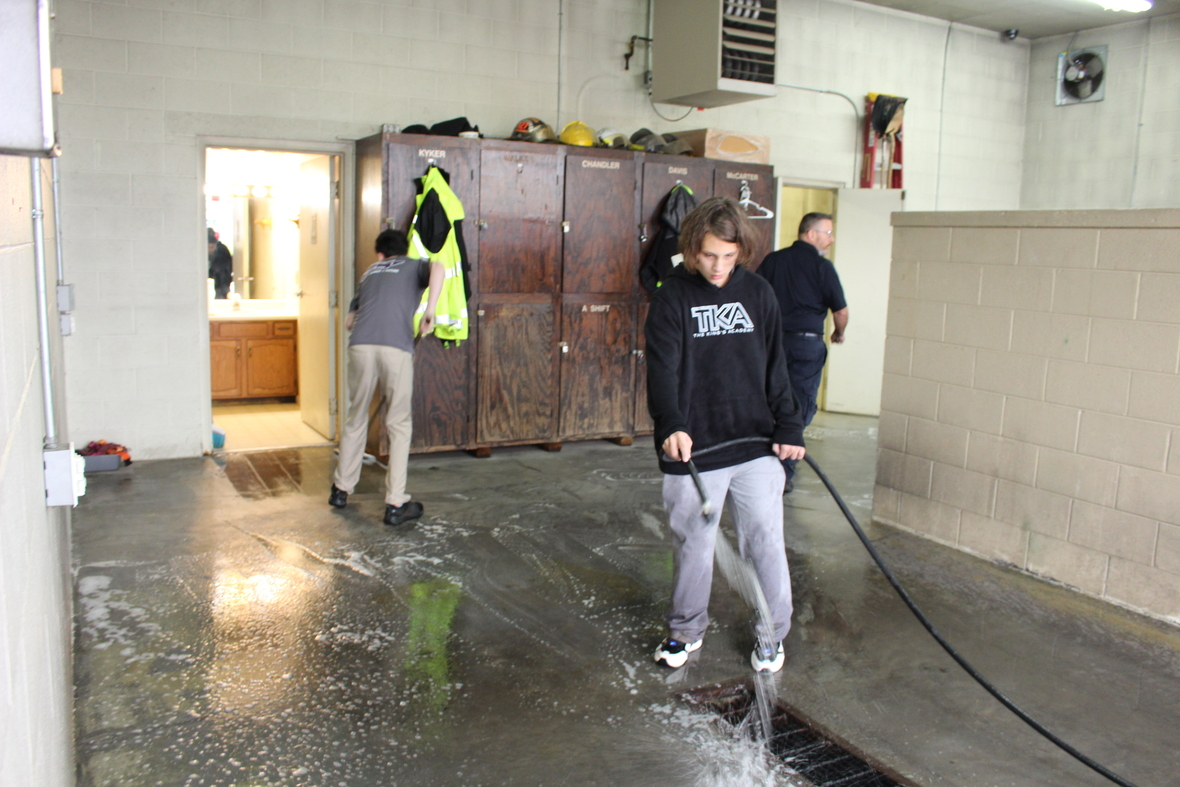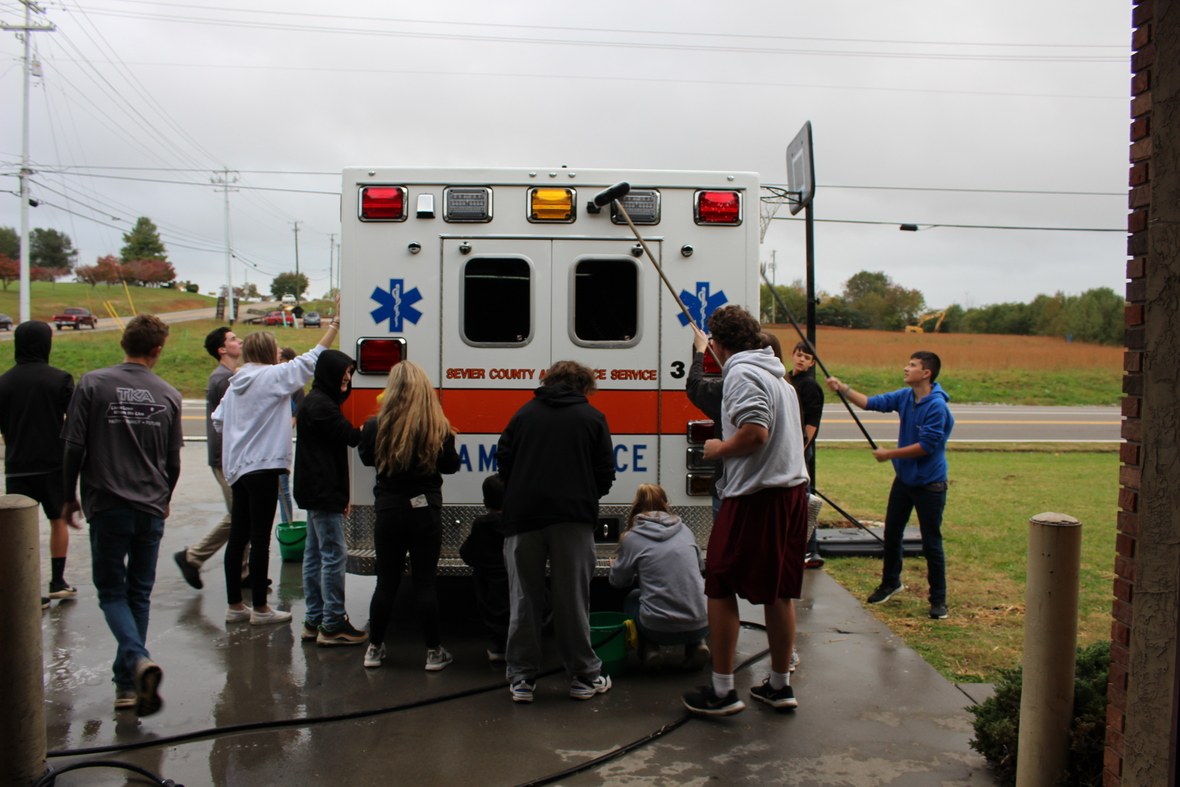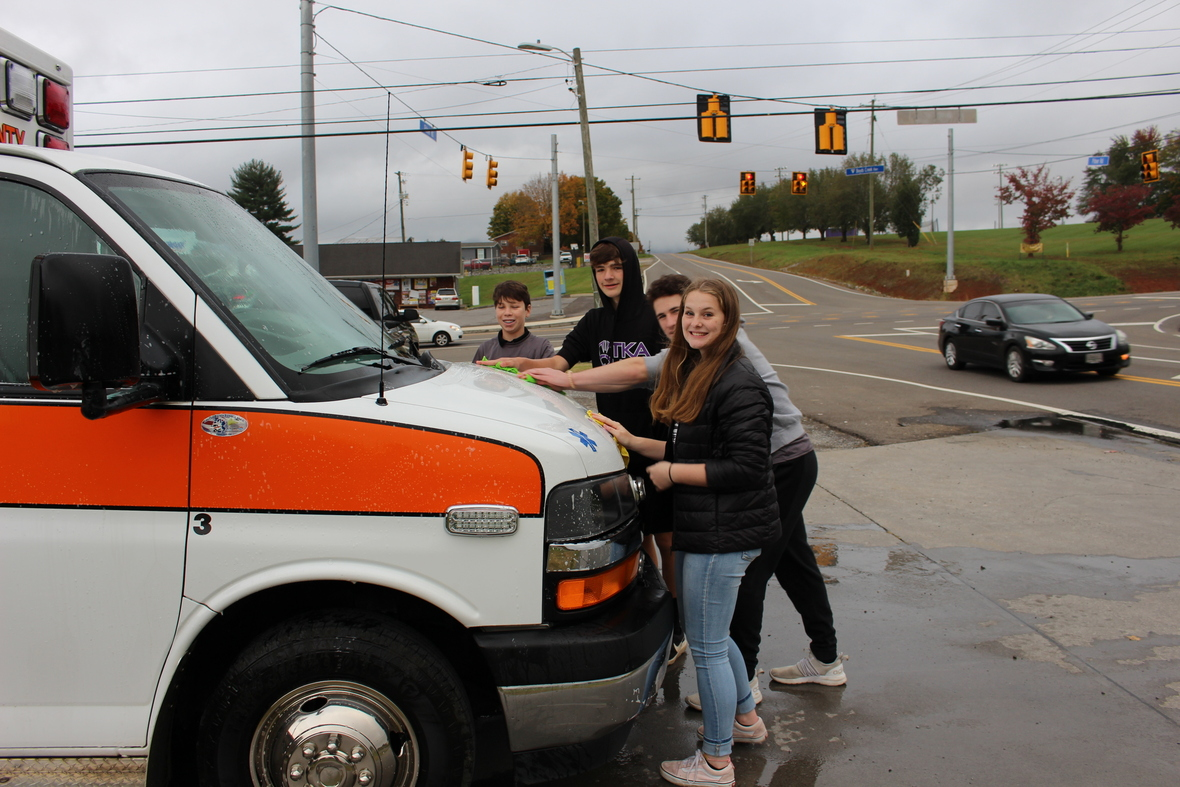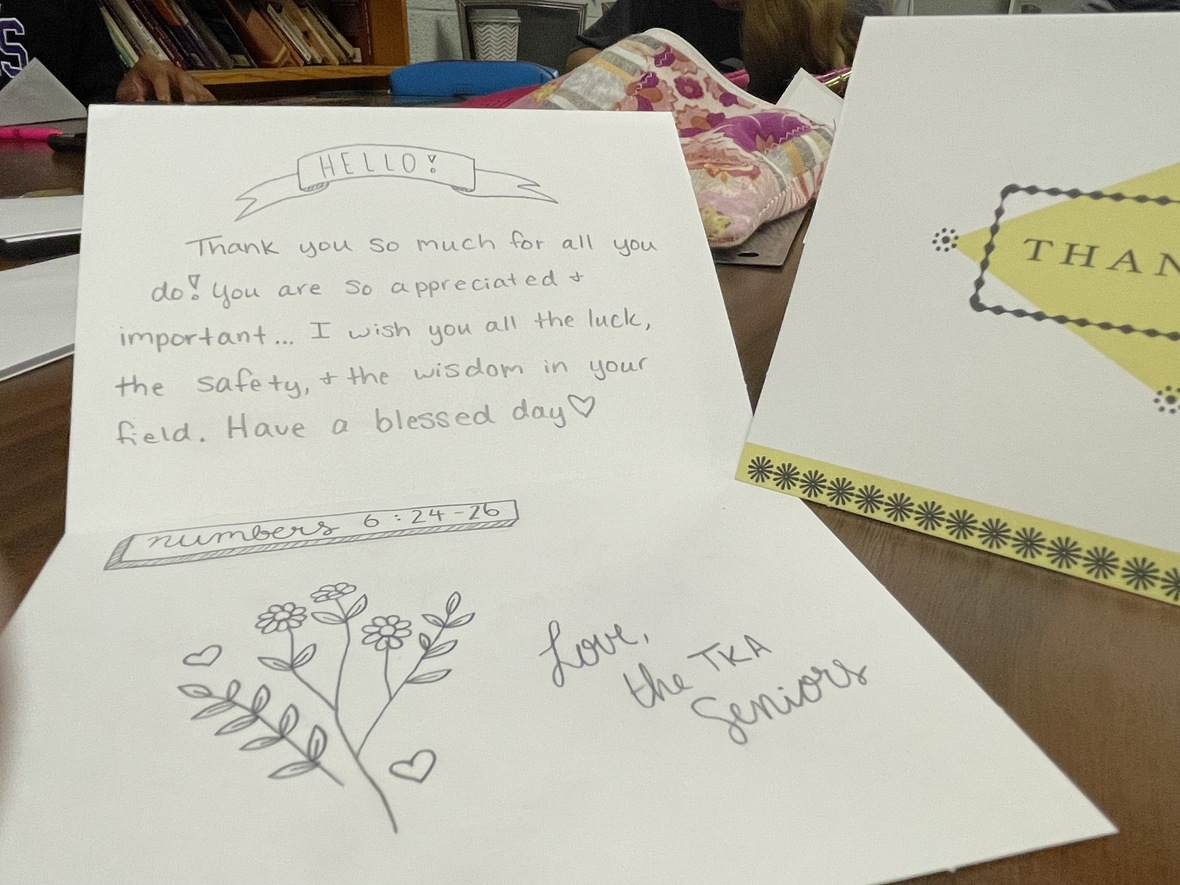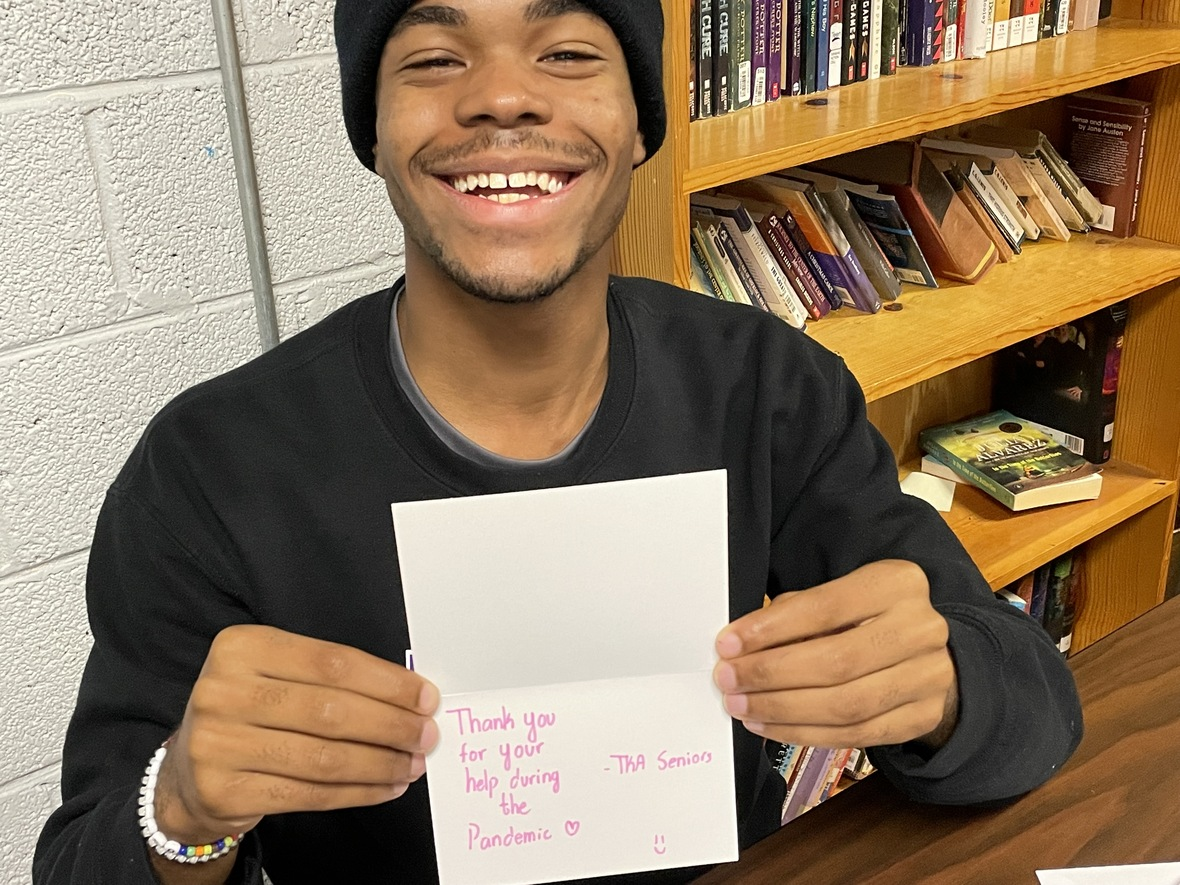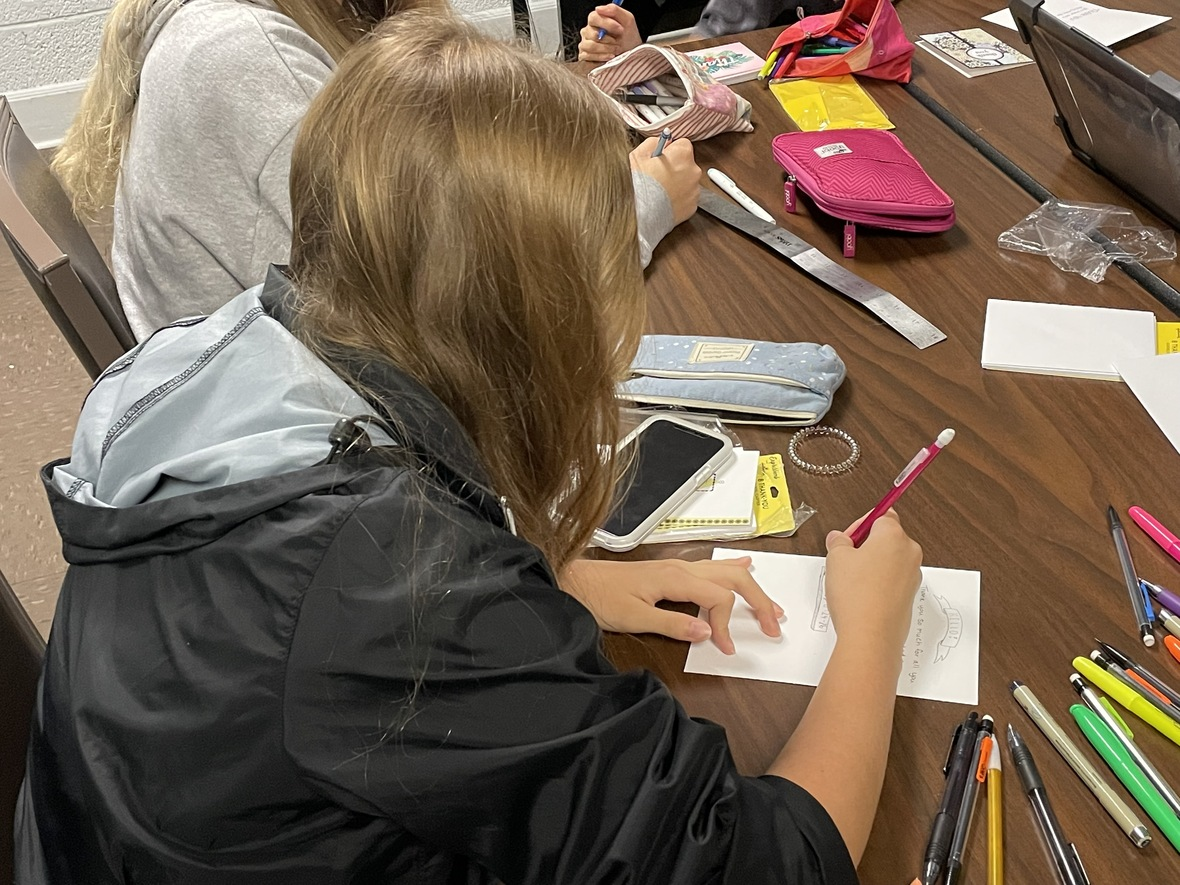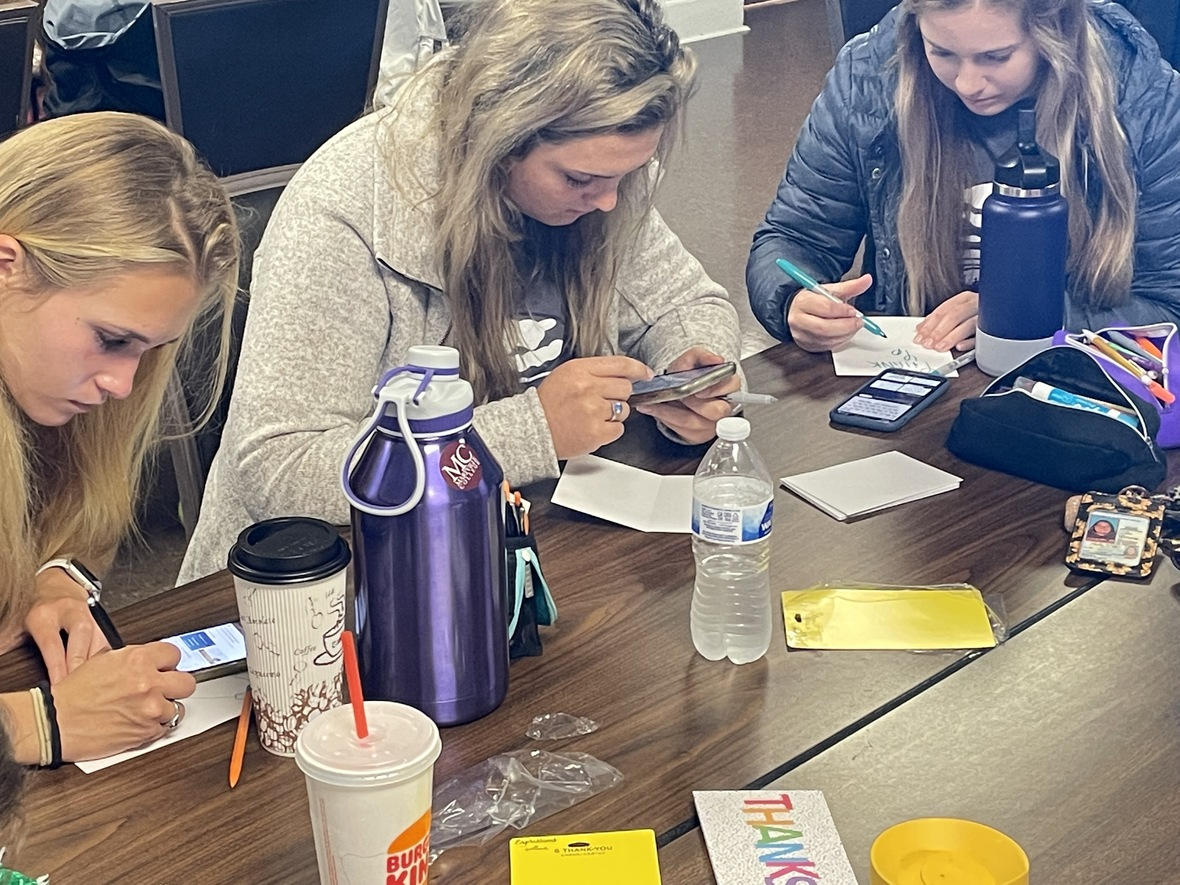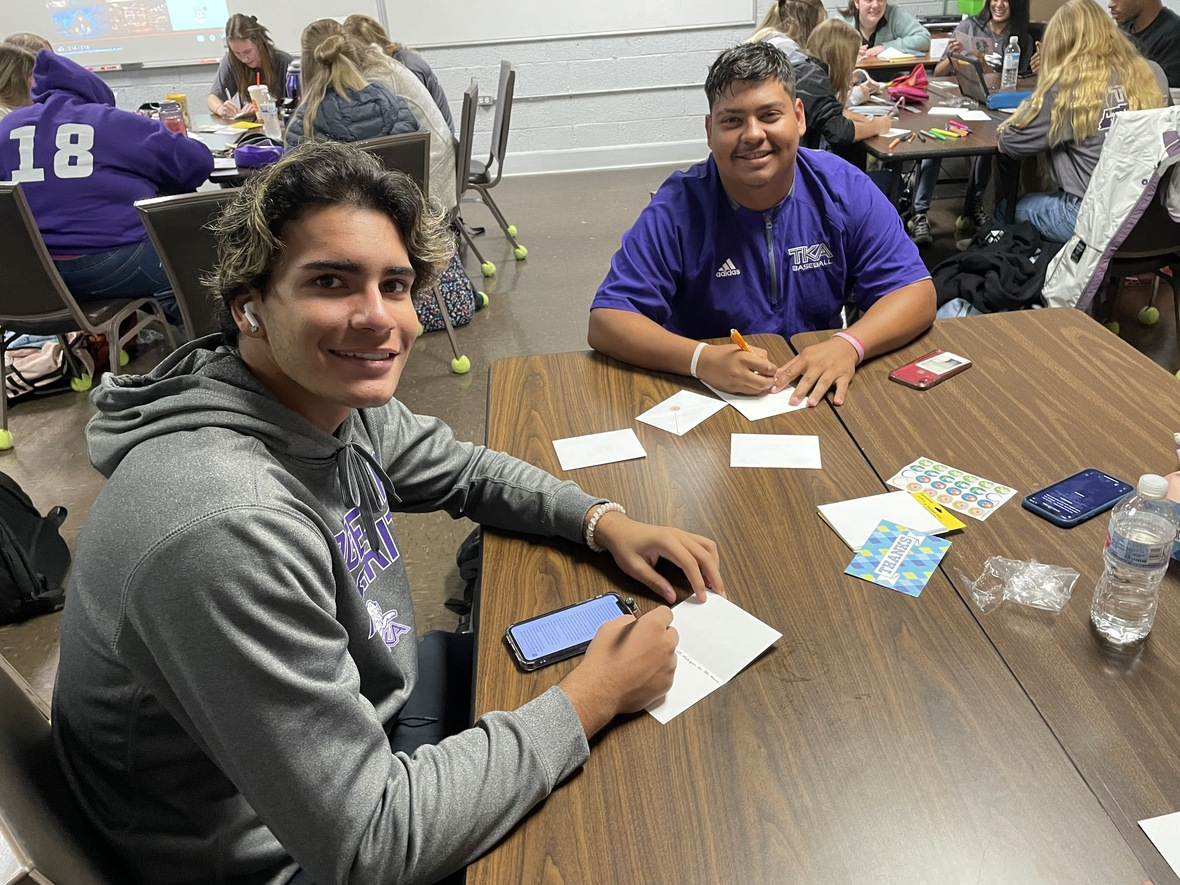table div table+table+table+table+table+table+table+table+table+table+table+table+table+table+table+table+table+table+table+table+table div table{width:100%;padding:0}table div table+table+table+table+table+table+table+table+table+table+table+table+table+table+table+table+table+table+table+table+table div table img{width:96.23%;padding:0;float:none}table div table+table+table+table+table+table+table+table+table+table+table+table+table+table+table+table+table+table+table+table+table div table td{width:100%;padding:0 1.88% 18px}/* styles */# National Principals Month

October is National Principals Month. We want to take time and celebrate our two TKA Principals! Mr. Fuller is our middle and high school principal, and Mrs. Sullivan oversees our elementary department as their principal.

Mr. Fuller received his B.A., Psychology, and M.Ed. in Educational Leadership from Saint Leo University. He has been serving at TKA since 2019.

Mrs. Sullivan received her B.S. in Elementary Education from Tennessee Wesleyan. She received her M.Ed. in Curriculum and Instruction and Ed.S in Administration and Supervision from Lincoln Memorial University. Mrs. Sullivan has been on staff at TKA since 2018.

Both principals go above and beyond each day to serve our students, teachers, and families. We at TKA are thankful for Mr. Fuller and Mrs. Sullivan! If you pass them in the hallways, give them a high five, fist bump, or even a hug to thank them for all they do daily to help our TKA Lions!table div table+table+table+table+table+table+table+table+table+table+table+table+table+table+table+table+table+table+table+table+table+table+table+table div table{width:100%;padding:0}table div table+table+table+table+table+table+table+table+table+table+table+table+table+table+table+table+table+table+table+table+table+table+table+table div table img{width:96.23%;padding:0;float:none}table div table+table+table+table+table+table+table+table+table+table+table+table+table+table+table+table+table+table+table+table+table+table+table+table div table td{width:100%;padding:0 1.88% 18px}/* styles */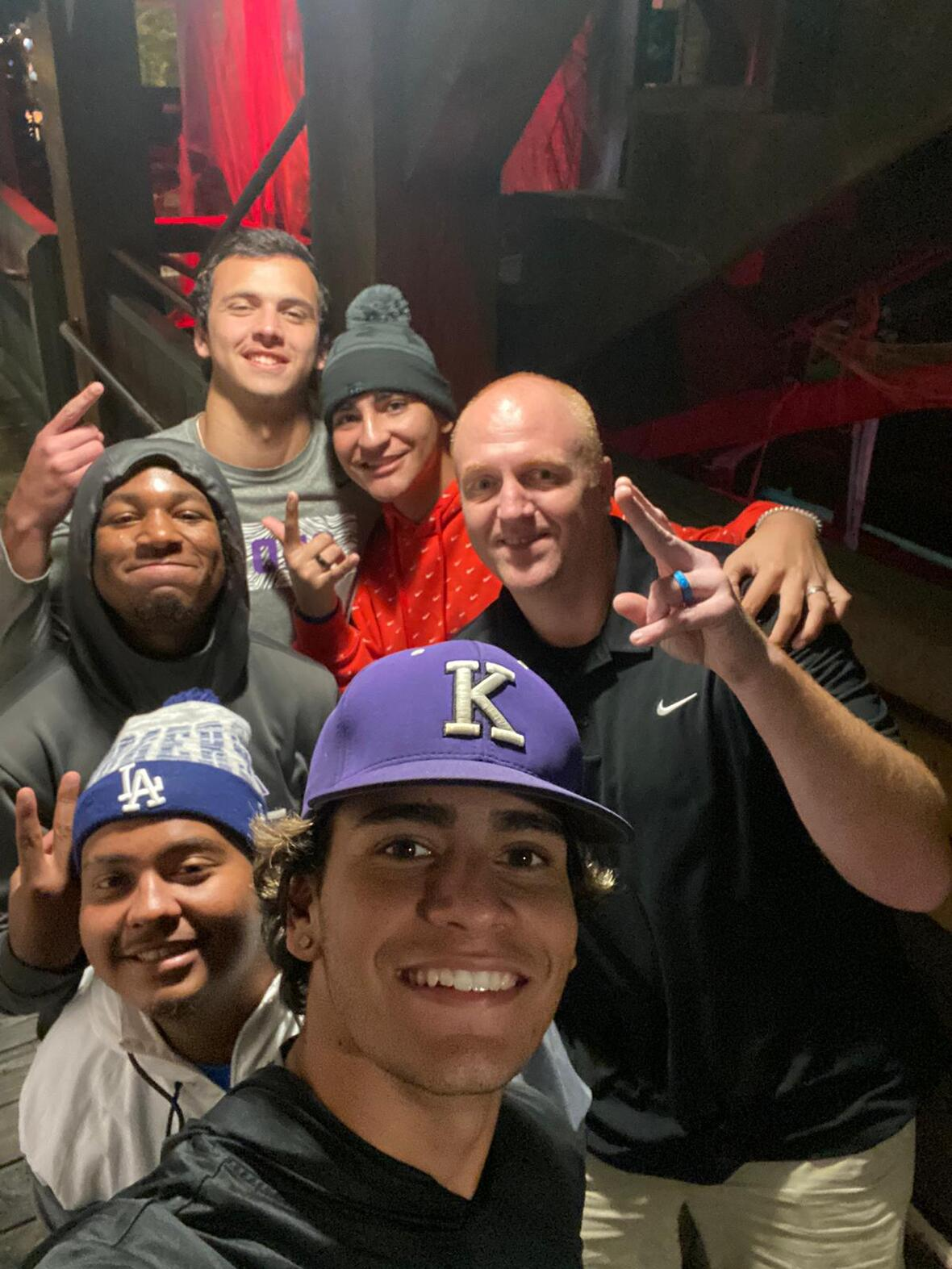# Residence Life in Action

This week, a few of our residence life students went to visit Ripley's Haunted Adventure. We love to see our dorm students having fun in the community while exploring where we live!

 table div table+table+table+table+table+table+table+table+table+table+table+table+table+table+table+table+table+table+table+table+table+table+table+table+table+table div table{width:100%;padding:0}table div table+table+table+table+table+table+table+table+table+table+table+table+table+table+table+table+table+table+table+table+table+table+table+table+table+table div table img{width:96.23%;padding:0;float:none}table div table+table+table+table+table+table+table+table+table+table+table+table+table+table+table+table+table+table+table+table+table+table+table+table+table+table div table td{width:100%;padding:0 1.88% 18px}/* styles */table div table+table+table+table+table+table+table+table+table+table+table+table+table+table+table+table+table+table+table+table+table+table+table+table+table+table+table+table div table{width:100%;padding:0}table div table+table+table+table+table+table+table+table+table+table+table+table+table+table+table+table+table+table+table+table+table+table+table+table+table+table+table+table div table img{width:96.23%;padding:0;float:none}table div table+table+table+table+table+table+table+table+table+table+table+table+table+table+table+table+table+table+table+table+table+table+table+table+table+table+table+table div table td{width:100%;padding:0 1.88% 18px}/* styles */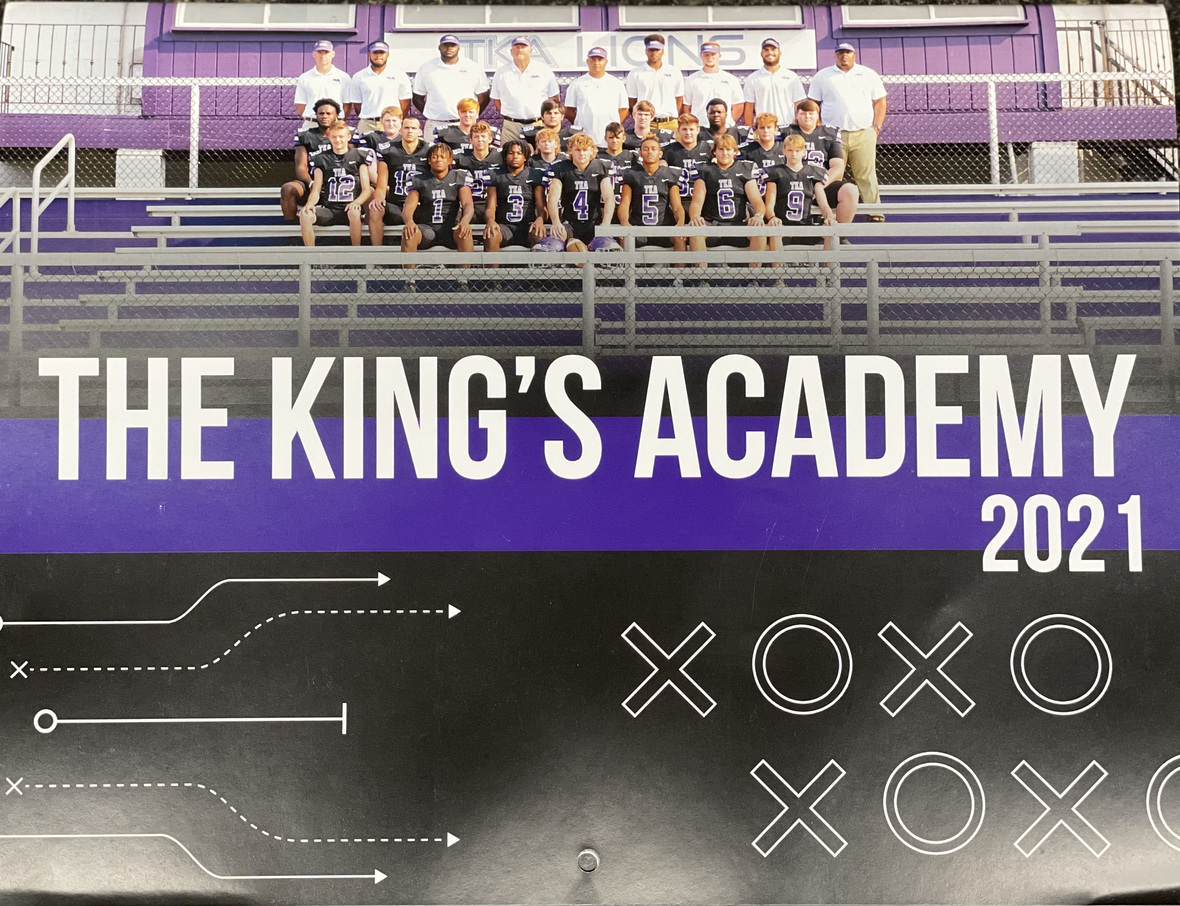# 2021-2022 Football Calendar

This year's football calendars are for sale for \$10! Stop by the school office to pick up your copy today!

 table div table+table+table+table+table+table+table+table+table+table+table+table+table+table+table+table+table+table+table+table+table+table+table+table+table+table+table+table+table+table div table{width:100%;padding:0}table div table+table+table+table+table+table+table+table+table+table+table+table+table+table+table+table+table+table+table+table+table+table+table+table+table+table+table+table+table+table div table img{width:96.23%;padding:0;float:none}table div table+table+table+table+table+table+table+table+table+table+table+table+table+table+table+table+table+table+table+table+table+table+table+table+table+table+table+table+table+table div table td{width:100%;padding:0 1.88% 18px}/* styles */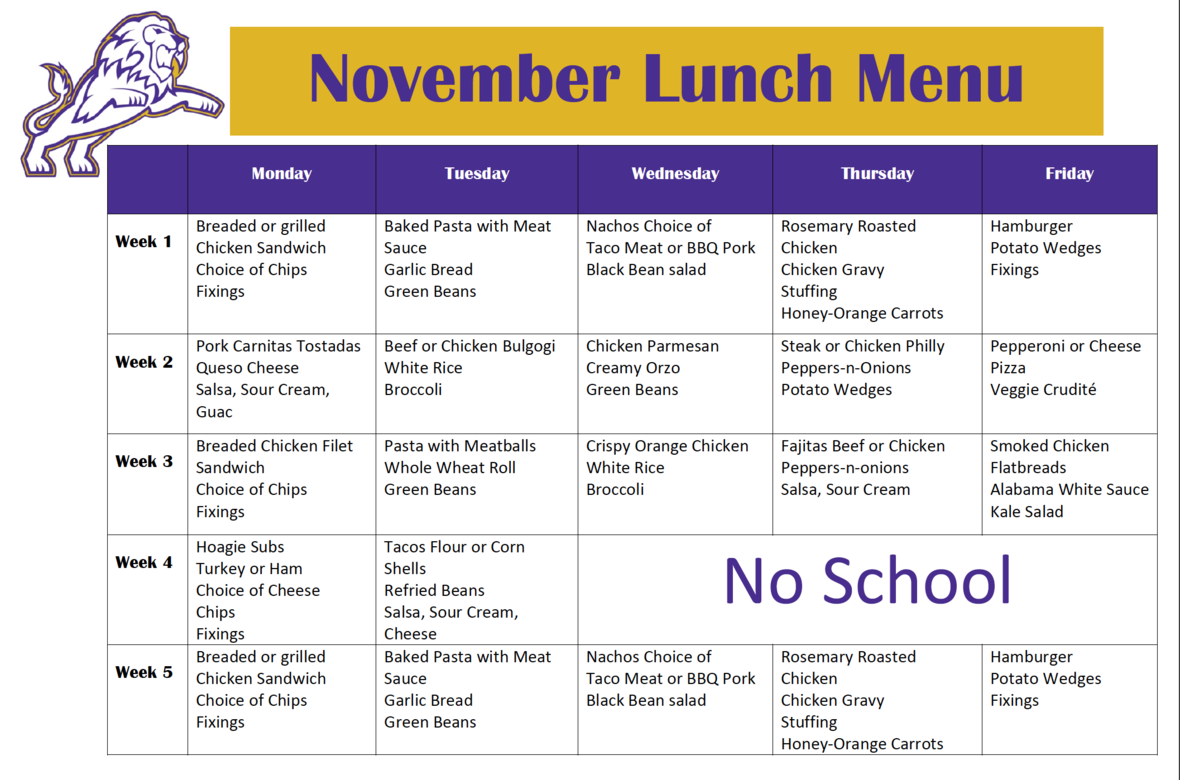table div table+table+table+table+table+table+table+table+table+table+table+table+table+table+table+table+table+table+table+table+table+table+table+table+table+table+table+table+table+table+table+table div table{width:100%;padding:0}table div table+table+table+table+table+table+table+table+table+table+table+table+table+table+table+table+table+table+table+table+table+table+table+table+table+table+table+table+table+table+table+table div table img{width:96.23%;padding:0;float:none}table div table+table+table+table+table+table+table+table+table+table+table+table+table+table+table+table+table+table+table+table+table+table+table+table+table+table+table+table+table+table+table+table div table td{width:100%;padding:0 1.88% 18px}/* styles */# Covid-19 Procedures

While we are excited to offer in-person education, we must continue to maintain a safe learning environment for all students, faculty/staff, and additional stakeholders.

To do so, we will be working closely with the Sevier County Health Department in regards to Covid-19 protocols.

 table div table+table+table+table+table+table+table+table+table+table+table+table+table+table+table+table+table+table+table+table+table+table+table+table+table+table+table+table+table+table+table+table+table+table div table{width:100%;padding:0}table div table+table+table+table+table+table+table+table+table+table+table+table+table+table+table+table+table+table+table+table+table+table+table+table+table+table+table+table+table+table+table+table+table+table div table img{width:96.23%;padding:0;float:none}table div table+table+table+table+table+table+table+table+table+table+table+table+table+table+table+table+table+table+table+table+table+table+table+table+table+table+table+table+table+table+table+table+table+table div table td{width:100%;padding:0 1.88% 18px}/* styles */table div table+table+table+table+table+table+table+table+table+table+table+table+table+table+table+table+table+table+table+table+table+table+table+table+table+table+table+table+table+table+table+table+table+table+table+table div table{width:100%;padding:0}table div table+table+table+table+table+table+table+table+table+table+table+table+table+table+table+table+table+table+table+table+table+table+table+table+table+table+table+table+table+table+table+table+table+table+table+table div table img{width:96.23%;padding:0;float:none}table div table+table+table+table+table+table+table+table+table+table+table+table+table+table+table+table+table+table+table+table+table+table+table+table+table+table+table+table+table+table+table+table+table+table+table+table div table td{width:100%;padding:0 1.88% 18px}/* styles */